# SAT Math Multiple Choice Question 448: Answer and Explanation

### Test Information

Question: 448

13. If h is a rational number such that –1 < h < 0, which of the following could be the graph of the equation y = hy + hx + x – 4?

• A.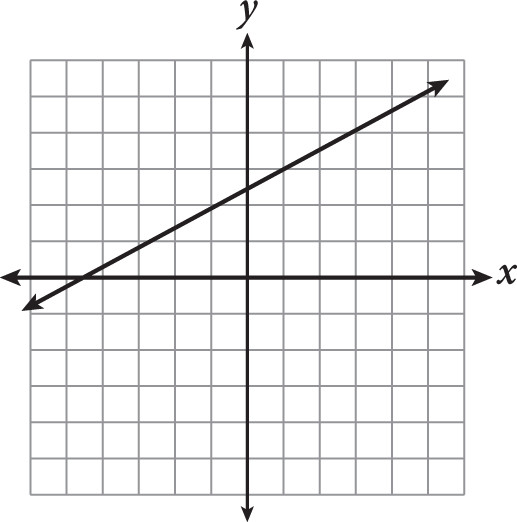• B.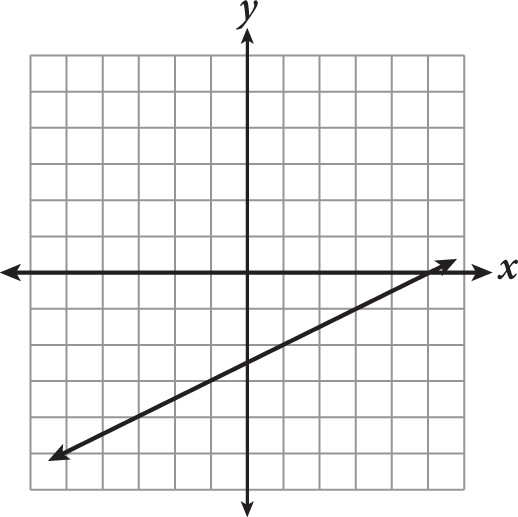• C.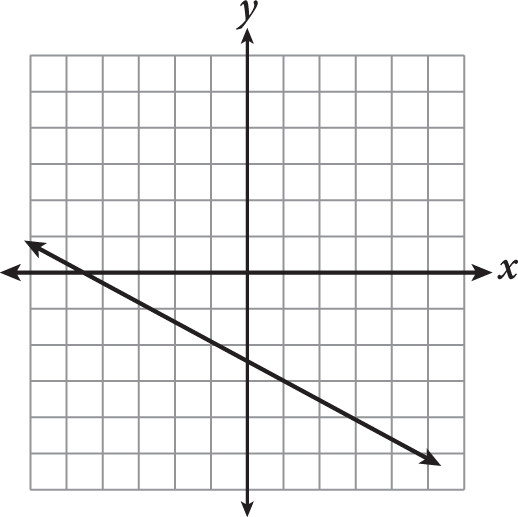• D.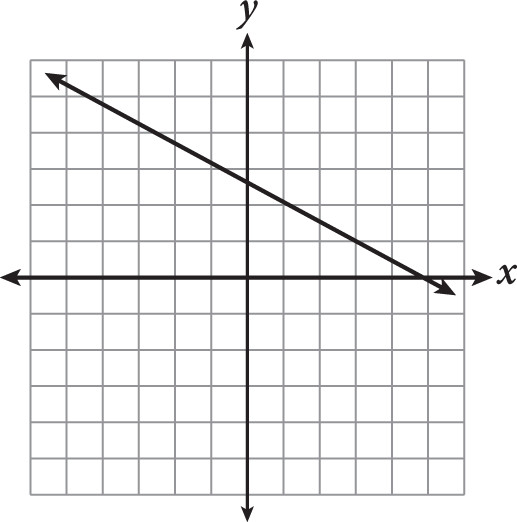Explanation:

B

Difficulty: Hard

Category: Heart of Algebra / Linear Equations

Strategic Advice: None of the variables in the equation has an exponent greater than 1, so the equation is linear. Most of what we know about lines revolves around slope and y-intercepts, so start by rearranging the equation to look like y = mx + b.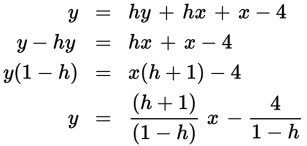Once rewritten, you have a linear equation with a slope of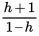and a y-intercept of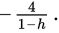It is given in the question that –1 < h < 0 (or a negative fraction greater than –1), so the quantity h + 1 is positive and the quantity 1 – h is also positive, resulting in a positive slope and a negative y-intercept. Only (B) satisfies these conditions.